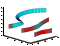# streamribbon## 语法

```streamribbon(X,Y,Z,U,V,W,startx,starty,startz) streamribbon(U,V,W,startx,starty,startz) streamribbon(vertices,X,Y,Z,cav,speed) streamribbon(vertices,cav,speed) streamribbon(vertices,twistangle) streamribbon(...,width) streamribbon(axes_handle,...) h = streamribbon(...) ```

## 说明

`streamribbon(X,Y,Z,U,V,W,startx,starty,startz)` 从向量三维体数据 `U``V``W` 绘制流带。

`startx`, `starty``startz` 定义流带的起始位置（中心处）。

`streamribbon(U,V,W,startx,starty,startz)` 假定 `X``Y``Z` 由以下表达式确定

```[X,Y,Z] = meshgrid(1:n,1:m,1:p) ```

`streamribbon(vertices,X,Y,Z,cav,speed)` 使用预先计算的流线图顶点、旋转角速度和流速。`vertices` 是流线图顶点的元胞数组（就像由 `stream3` 生成一样）。`X``Y``Z``cav``speed` 是三维数组。

`streamribbon(vertices,cav,speed)` 假定 `X``Y``Z` 由以下表达式确定

```[X,Y,Z] = meshgrid(1:n,1:m,1:p) ```

`streamribbon(vertices,twistangle)` 将包含向量 `twistangle` 的元胞数组用于条带的扭曲度（以弧度为单位）。`vertices``twistangle` 的每个对应元素的大小必须相等。

`streamribbon(...,width)` 将条带的宽度设置为 `width`

`streamribbon(axes_handle,...)` 将图形绘制到句柄为 `axes_handle` 的坐标区对象中，而不是当前坐标区对象 (`gca`) 中。

`h = streamribbon(...)` 将句柄（每个起始点一个句柄）向量返回到 `surface` 对象。

## 示例

```load wind [sx,sy,sz] = meshgrid(80,20:10:50,0:5:15); streamribbon(x,y,z,u,v,w,sx,sy,sz); axis tight shading interp view(3); camlight lighting gouraud``````load wind [sx,sy,sz] = meshgrid(80,20:10:50,0:5:15); verts = stream3(x,y,z,u,v,w,sx,sy,sz); cav = curl(x,y,z,u,v,w); spd = sqrt(u.^2 + v.^2 + w.^2).*.1; streamribbon(verts,x,y,z,cav,spd); axis tight shading interp view(3); camlight; lighting gouraud``````t = 0:.15:15; verts = {[cos(t)' sin(t)' (t/3)']}; twistangle = {cos(t)'}; streamribbon(verts,twistangle); axis tight shading interp view(3) camlight lighting gouraud``````xmin = -7; xmax = 7; ymin = -7; ymax = 7; zmin = -7; zmax = 7; x = linspace(xmin,xmax,30); y = linspace(ymin,ymax,20); z = linspace(zmin,zmax,20); [x,y,z] = meshgrid(x,y,z); u = y; v = -x; w = 0*x+1; [cx,cy,cz] = meshgrid(linspace(xmin,xmax,30),... linspace(ymin,ymax,30),[-3 4]); h = coneplot(x,y,z,u,v,w,cx,cy,cz,'quiver'); set(h,'Color','k');``````[sx,sy,sz] = meshgrid([-1 0 1],[-1 0 1],-6); streamribbon(x,y,z,u,v,w,sx,sy,sz); [sx,sy,sz] = meshgrid([1:6],,-6); streamribbon(x,y,z,u,v,w,sx,sy,sz); shading interp view(-30,10) axis off tight camproj perspective camva(66) camlookat camdolly(0,0,.5,'fixtarget') camlight```